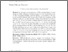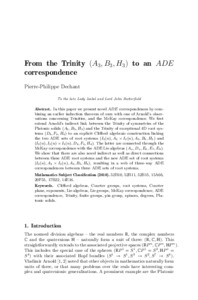# From the Trinity $(A_3, B_3, H_3)$ to an $ADE$ correspondence

(2018) From the Trinity $(A_3, B_3, H_3)$ to an $ADE$ correspondence. Proceedings of the Royal Society A: Mathematical, Physical and Engineering Sciences, 474 (2220).Preview
Text
PPD_Trinity.pdf - Accepted Version
In this paper we present novel $ADE$ correspondences by combining an earlier induction theorem of ours with one of Arnold's observations concerning Trinities, and the McKay correspondence.
We first extend Arnold's indirect link between the Trinity of symmetries of the Platonic solids $(A_3, B_3, H_3)$ and the Trinity of exceptional 4D root systems $(D_4, F_4, H_4)$ to an explicit Clifford algebraic construction linking the two ADE sets of root systems $(I_2(n), A_1\times I_2(n), A_3, B_3, H_3)$ and $(I_2(n), I_2(n)\times I_2(n), D_4, F_4, H_4)$.
The latter are connected through the McKay correspondence with the ADE Lie algebras $(A_n, D_n, E_6, E_7, E_8)$. We show that there are also novel indirect as well as direct connections between these ADE root systems and the new ADE set of root systems $(I_2(n), A_1\times I_2(n), A_3, B_3, H_3)$, resulting in a web of three-way ADE correspondences between three ADE sets of root systems.